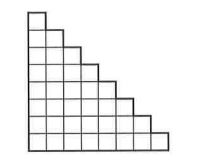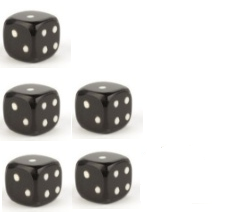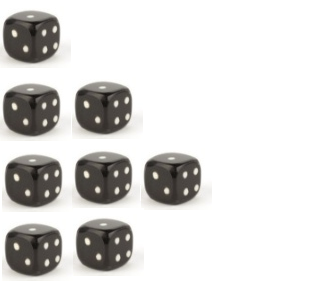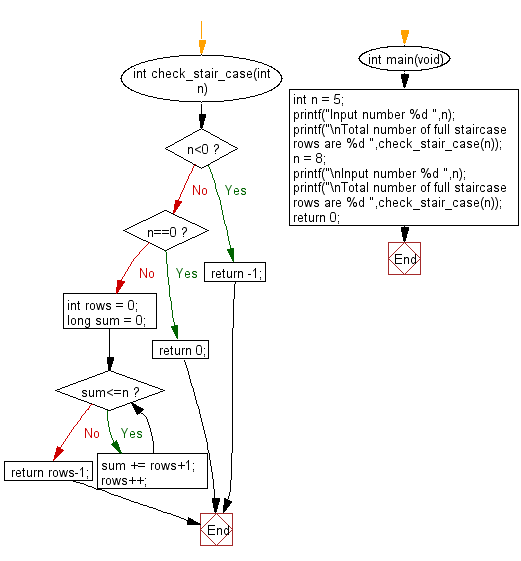﻿ C Program: Full staircase rows built from a dice

# C Exercises: Find the total number of full staircase rows that can be formed from given number of dices

## C Programming Mathematics: Exercise-18 with Solution

Write a C program to find the total number of full staircase rows that can be formed from a given number of dice.

staircase rows format :Example 1:
n = 5
The dices can form the following rows:As the 3rd row is incomplete the program will return 2 (full staircase rows).
Example 2:
n = 8 The dices can form the following rows:As the 4th row is incomplete the program will return 3 (full staircase rows).

Sample Solution:

C Code:

``````#include <stdio.h>

int check_stair_case(int n) {
if(n<0) return -1;
if(n==0) return 0;
int rows = 0;
long sum = 0;
while(sum<=n){
sum += rows+1;
rows++;
}
return rows-1;
}

int main(void)
{
int n = 5;
printf("Input number %d ",n);
printf("\nTotal number of full staircase rows are %d ",check_stair_case(n));
n = 8;
printf("\nInput number %d ",n);
printf("\nTotal number of full staircase rows are %d ",check_stair_case(n));
return 0;
}
```
```

Sample Output:

```Input number 5
Total number of full staircase rows are 2
Input number 8
Total number of full staircase rows are 3
```

Flowchart:C Programming Code Editor:

Improve this sample solution and post your code through Disqus.

What is the difficulty level of this exercise?

Test your Programming skills with w3resource's quiz.

﻿